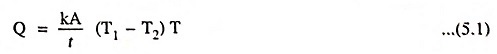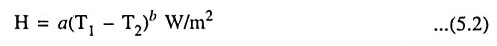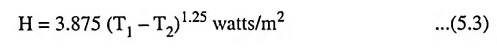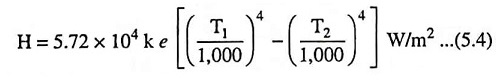## Three Modes of Heat Transfer (Conduction, Convection, Radiation):

A heated substance will give off heat to another substance at a lower temperature. The three different modes of heat transfer are

1. Conduction,
2. Convection and

### 1. Conduction:

In this modes of heat transfer, one molecule of the substance gets heated and transfer the heat to the adjacent one and so on. Thus heat is transferred through a substance from one part to another, or between two substances in contact.

The rate of conduction of heat along a substance depends upon the temperature gradient. It may be expressed in MJ per hour per square metre per metre or in watts per square centimetre per centimetre while dealing electric heating.

In a plate of thickness t metres, having x-sectional area of its two parallel faces A square metres and temperature of its two faces T1 and T2 °C absolute the quantity of heat passed through it during T hours is given bywhere

• k is the coefficient of thermal conductivity for the material in MJ/m2/m/°C/hour.

### 2. Convection:

Heat is transferred by convection in case of immersion type water heater or in case of low tempera­ture heating equipment for buildings. The air in contact with a heated radiator element in a room receives heat from con­tact with the element. The heated air expands and rises, cold air flowing into take its place. Thus there is a constant flow of air upwards across the heating element. This modes of heat transfer process is called convection. These convection currents give up some of their heat to the colder parts of the room. The room and its contents are thus gradually heated by this means. A simi­lar action takes place in an electric water heater, a continu­ous flow of water passing upwards across the immersed heater element, with the result that the whole of the water in the tank becomes hot.

The quantity of heat absorbed from the heater by convection depends mainly upon the temperature of the heating element above the surroundings and upon the size of the surface of the heater. It also depends partly on the position of the heater. Heat dissipation is given by the following expression.

Heat dissipation,where

• a and b are constants, whose value depend upon the heating surface facilities for heating etc.
• T1 and T2 are the temperatures of the heating surface and the fluid in °C absolute respectively.

For vertical surfaces in air, the above expression becomes

Heat dissipation,In furnaces heat transfer by convection is not to any great extent.

In this modes of heat transfer, the heat reaches the substance to be heated from the source of heat without heating the medium in between. Rate of heat radiation is given by Stefan’s law, according to which

Heat dissipation,where

• T1 is temperature of source of heat in °C absolute,
• T2 is temperature of substance to be heated in °C absolute,
• k is a constant known as radiant efficiency,

k is unity for a single element and between 0.5 and 0.8 for several elements placed side by side and e is emissivity, which is unity for black body and is equal to 0.9 for resistance heating elements.

Since radiation is proportional to the difference of fourth powers of temperatures, we can have very efficient heating at high temperatures.

Scroll to Top